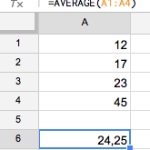# Calculate Averages in Excel

Discover how to find the average using this simple formula for Microsoft Excel, Discover the mean value of different figures with one single equation.The average is usually calculated by adding all the values together and dividing them by the total number of values that you have added. For example, if you have 3 different values, you can discover the average by adding them all together and dividing by 3:

`average = (value1 + value2 + value3) / 3`

## How to calculate the average in Excel

You can make this calculation even easier as there is a specific Excel function for this equation, the AVERAGE function:

`=AVERAGE(value1; [value2,...)`To calculate the average of several numbers all you have to do is insert the code AVERAGE and enter all the different values. Excel will automatically calculate the average from your figures. For example, if you insert 4 values into the first four cells (A1, A2, A3, A4) your formula will be:

`=AVERAGE(A1:A4)`